# Conditional Statements in C++

## Conditional Statements in C++ with Examples

To make our discussion interesting I am going to introduce one of the most important topics in any programming language which is Conditional Statements in C++ Language along with the Logical Operators and Relational Operators with Examples. Here, you will learn what is a conditional statement and what are the ways of writing them and will use them in various programs. So let us start with the C++ Conditional Statements.

##### Conditional Statements in C++:

In our daily life, we come across conditional processing in various places. For example, if you’re logging on to any website then you have to give your user ID and Password. If the credentials are valid then you will be allowed to enter into the otherwise else you will not allow.

So, the conditional statement comes into the picture when we have choices. To understand better what exactly a conditional statement is let us take some more examples:

1. Marks: Usually we consider marks above 35 to have passed and below 35 to have failed. Here we have the choice of pass or fail, which is decided based on marks scored.
2. Offers: If you purchase 4000 and above 10% discount else no discount. Again, here we have the choice of whether to do the shopping for more than 4000 to be eligible for a 10% discount.

So, like these examples, there are many places where we use if and else conditional statements. So, the same thing in computation we use conditional statements. From the above examples, we can say that usually conditional statements have two states true or false. The true state in programming is 1 and the False state is 0.

##### Examples of true state and false state:

If (marks greater than 35)
Pass //This block is an example of a True state
Else
Fail //This block is an example of a False state.

##### How to Write a Conditional Statement in C++?

So let us see how to write a conditional statement in C++ and how they work.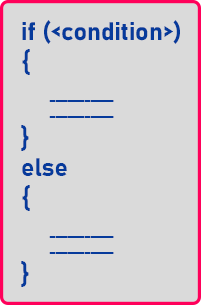This is a skeleton of a C++ conditional statement. We need to provide the condition inside if (_condition_) and then if the condition is true then the if block will be executed. And if the given condition is false then the else block will be executed. So, depending on the result of the condition either the if block is executed or the else block is going to be executed.

##### Flowchart of IF Conditional Statement:

The following diagram represents the flow chart for the ‘if’ conditional statement.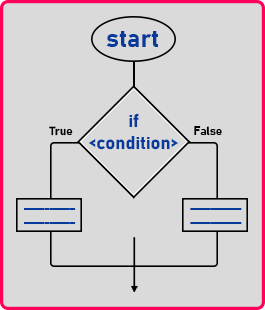As you can see in the above image, the square part is used for checking the condition. If the condition is true then the left block of statements will be executed and then it will continue further its execution. On the other hand, if the condition is false, then it will branch in the right direction, and it will execute the false block, and then continue further its execution. So, this shows the flow of control in the case of ‘if’ and ‘else’. So, this is the syntax of a conditional statement and one more point that you need to remember is the else part is optional.

##### What is this true and false?

The next thing that we will learn is what is true and false. In C++ programming, false means ‘0’ and any other value means true. So usually we say that ‘1’ means true, actually not just 1 but any other number of integers type we considered as true. So, you can write true and false or even you can use 0 and 1 in C++.

##### How to Write Conditions in C++?

Now, the next thing that we will learn is how to write this condition. In C++, the conditions are written using relational operators. We write conditional statements using relational operators and also logical operators. In our upcoming articles, we will discuss Logical operators. So, let us first discuss something about Relational operators, and then we will discuss how to write conditional statements using Relational operators in C++.

##### Relational Operator in C++

As the name itself suggest Relational operators are used to finding the relation between two operands. Relational Operators in C++ are as follows.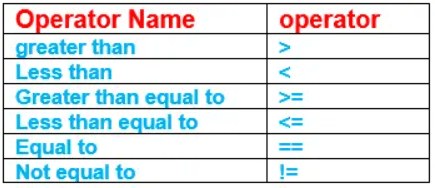Before talking more about Relational operators and their relation with conditional statements, let’s talk about something which we usually discuss in the case of operators. Yes, you guessed it right…! Relational operators Precedence and Associativity.

##### Relational Operator Precedence and Associativity:

Relation operator <, >, <=, >= have same precedence whereas == and != has relatively lower precedence but all relational operators have left to right associativity.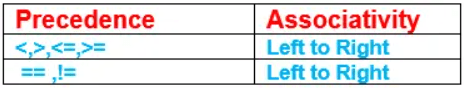Note Arithmetic operators as a higher precedence than Relational operators.

##### Relation Between Conditional Statements and Relational Operators in C++

The Relation operators are used for comparing the values on either side. Usually, conditions are evaluated based on comparison and hence both Relational Operators and Conditional Statements have tight coupling as both go together.

Example: marks>35 here > is a relational operator. Let us dive into the first conditional statement:

##### Conditional if Statement in C++:

In conditional if, the task is performed only if the condition is true. And the false condition is unhandled.

###### General Syntax:

if(condition){
//logic that needs to implements if true.
}

For better understanding, please have a look at the below code.

int a = 10, b = 15;
if (a < b){
cout << a << endl;
}

Here we have two variables ‘a’ and ‘b’. We have assigned some value to both of them. Next inside ‘if’, we are checking for ‘a < b’. In this case, yes, ‘a’ is smaller than ‘b’ then it goes to the ‘if’ block where we are printing the value of the ‘a’ variable.

##### If Conditional Statements Example in C++ Language:

In the below example print of a happens only when a is 10. Here the other value of a is unhandled. Here == is a relational operator.

```#include <iostream>
using namespace std;
int main ()
{
int a = 10;
if (a == 10)
{
cout << "The value of a is 10\n" << endl;
}
return 0;
}
```

Output: The value of a is 10

##### If-Else Statement in C++ Language:

As the name suggests here, we handled both the conditions. True and false.
General Syntax of If-Else Conditional Statement is given below:
if(condition)
{
//logic when true;
}
else{
//logic when false;
}

##### Roll No. Validation Program in C++ using Conditional Statement:
```#include <iostream>
using namespace std;
int main()
{
int roll;
cout << "Enter your Roll No." << endl;
cin >> roll;
if (roll > 0)
{
cout << "Valid Roll No." << endl;
}
else
{
cout << "Invalid Roll No." << endl;
}
return 0;
}
```
###### Output: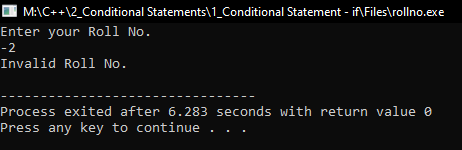##### Denominator Validation Program in C++ using Conditional Statement:
```#include <iostream>
using namespace std;
int main()
{
int a, b, c;
cout << "Enter two numbers: " << endl;
cin >> a >> b;

if (b == 0)
{
cout << "Invalid denominator" << endl;
}
else
{
c = a / b;
cout << c << endl;
}

return 0;
}
```
###### Output: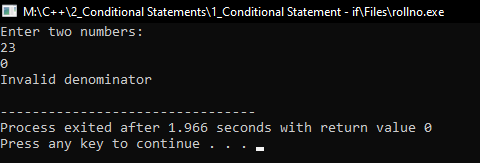In the next article, I am going to discuss How to Find Maximum of Two Numbers Program in C++ with Examples. Here, in this article, I try to explain Conditional Statements in C++ with Examples and I hope you enjoy this Conditional Statement in C++ with Examples article.